Home > CCA2 > Chapter 8 > Lesson 8.1.1 > Problem8-9

8-9.
1. Polynomials are expressions that can be written as a sum of terms of the form: (any number). x(whole number)

2. Which of the following equations are polynomial equations? For those that are not polynomials, explain why not. Check the Lesson 8.1.1 Math Notes box for further details about polynomials. Homework Help ✎

1. f(x) = 8x5 + x2 + 6.5x4 + 6

2. y =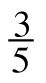x6 + 19x2

3. y = 2x + 8

4. f(x) = 9 + √x − 3

5. P(x) = 7(x − 3)(x + 5)2

6. y = x2 +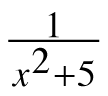7. Write an equation for a new polynomial function and then write an equation for a new function that is not a polynomial.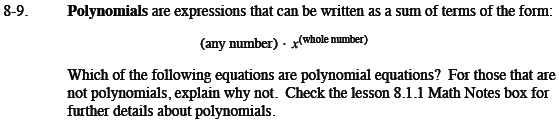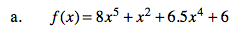This is a polynomial.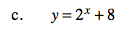This is not a polynomial because x is an exponent.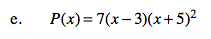This is a polynomial.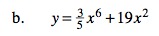This is a polynomial.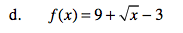This is not a polynomial becausethe power of x is a fraction, not a whole number.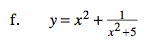This is not a polynomial becausethe variable is in the denominator.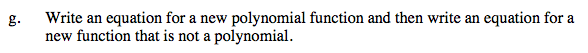Look at parts (a) through (f) for examples.